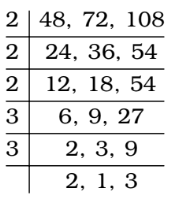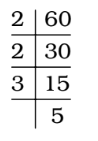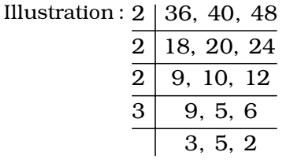## LCM and HCF

#### LCM and HCF

1. The maximum number of students among whom 1001 pens and 910 pencils can be distributed in such a way that each student gets same number of pens and same number of pencils, is :

1. As we know that ,
Maximum number of students = The greatest common divisor = HCF of 1001 and 910

##### Correct Option: A

As we know that ,
Maximum number of students = The greatest common divisor = HCF of 1001 and 910 = 91

1. Three electronic devices make a beep after every 48 seconds, 72 seconds and 108 seconds respectively. They beeped together at 10 a.m. The time when they will next make a beep together at the earliest is

1. Required time = LCM of 48, 72 and 108 seconds∴ LCM = 2 × 2 × 2 × 2 × 3 × 3 × 3 = 432 seconds = 7 minutes 12 second

##### Correct Option: A

Required time = LCM of 48, 72 and 108 seconds∴ LCM = 2 × 2 × 2 × 2 × 3 × 3 × 3 = 432 seconds = 7 minutes 12 second
∴ Required time = 10 a.m. + 7 minutes 12 second = 10 : 07 : 12 hours

1. The LCM fo two prime numbers p and q, (p > q) is 161. The value of (3q – p) :

1. LCM of p and q = 161
∴ pq = 23 × 7
∴ p = 23; q = 7
∴ 3q – p = 3 × 7 – 23

##### Correct Option: A

LCM of p and q = 161
∴ pq = 23 × 7
∴ p = 23; q = 7
∴ 3q – p = 3 × 7 – 23
The value of (3q – p) = 21 – 23 = – 2

1. The LCM of four consecutive numbers is 60. The sum of the first two numbers is equal to the fourth number. What is the sum of four numbers?

1. We find LCM of 60 ,∴ 60 = 2 × 2 × 3 × 5
i.e., Numbers = 2, 3, 4 and 5

##### Correct Option: B

We find LCM of 60 ,∴ 60 = 2 × 2 × 3 × 5
i.e., Numbers = 2, 3, 4 and 5
∴ Required sum = 2 + 3 + 4 + 5 = 14

1. Three bells ring at intervals of 36 seconds, 40 seconds and 48 seconds respectively. They start ringing together at a particular time. They will ring together after every

1. Required answer = LCM of 36, 40 and 48 seconds∴ LCM = 2 × 2 × 2 × 2 × 3 × 3 × 5 = 720

##### Correct Option: B

Required answer = LCM of 36, 40 and 48 seconds
= 720 seconds

 Required answer = 720 minutes = 12 minutes 60∴ LCM = 2 × 2 × 2 × 2 × 3 × 3 × 5 = 720